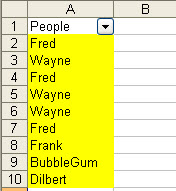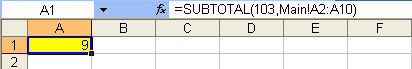# Trapping a change to a filtered list with VBA

Published on
14,253 Points
5,853 Views
4 Endorsements
Approved
Community Pick

## Introduction

This article provides a solution for the automatic detection of a change to the filtered list, and an altenative approach for using WorkSheet rather than Workbook Calculation settings.

In http://www.experts-exchange.com/Q_24097818.html) the Asker wanted to automatically trap a change to the filtering of a range. This question posed two issues:
1) the key problem is that Excel does not provide an inbuilt WorkSheet Event for filtering, so there is not an existing Event to do this.
2) a compounding factor is that for this particular Workbook, Calculation had been set to Manual given the spreadsheet complexity.

## Creating a "Range Filter" Event.

A change to a filtered list can be trapped with VBA with a simple two step workaround.
1) A "dummy" WorkSheet is added with a single SUBTOTAL formula in A1 pointing back to the range being filtered on the main sheet.

main sheet with list in A2:A10dummy sheet with SUBTOTAL formula in A1 pointing at the main sheet list in A2:A102) A Worksheet_Calculate() Event is added to the "dummy" WorkSheet, this Event fires when the SUBTOTAL formula updates when the filter is changed.

``````'Dummy sheet code
Private Sub Worksheet_Calculate()
'Dummy Sheet has recalculated
MsgBox "Your list has been filtered"
End Sub
``````

## Catering for Manual Calculation

Note that the approach above requires Workbook Calculation to be set to either Automatic (xlCalculationAutomatic in VBA), or Automatic except tables (xlCalculationSemiAutomatic). But in this particular question, the Asker had deliberately set Calculation to Manual (xlCalculationManual), so further coding was necessary to set the WorkBook up so that only the "dummy" WorkSheet would be set to automatically Calculate, all other sheets having Calculation turned off.

There is a rarely used WorkSheet property, EnableCalculation, that can be set via the Visual Basic Editor to True or False. The default setting is obviously True, if it is set to False then the worksheet will not calculate. The EnableCalculation property is not available to the regular Excel Menu or Ribbon options - so as an aside this can be a useful trick for people who are looking to secure Excel models by deliberately keeping key sheets from recalculating.

3) Add a Workbook_Open Event to set the EnableCalculation property of all sheets other than "Dummy" to False.
4) Run the Workbook in Calculation mode.

``````'ThisWorkbook Module
Option Explicit

Private Sub Workbook_Open()
Call AllButOne
End Sub

'Module 1
Sub AllButOne()
Dim ws As Worksheet
For Each ws In ThisWorkbook.Sheets
ws.EnableCalculation = (ws.Name = "Dummy")
Next ws
End Sub
``````

Attached is a sample file containing the example data and code referred to above.
24097818.xls

## Other

Please note that changes/updates to the list (deleting or adding entries) will also trigger a recalculation on the dummy sheet. If it is important to differentiate between a filtered change and a list change then the list contents will need to be stored and compared by the code.

## Appendix - Excel Events

Excel provides developers with automatic VBA trigger for "Events" on a WorkSheet, Workbook and Application level, the later being accessible via the use of class modules.

The WorkSheet Events include:
Activate

BeforeDoubleClick

BeforeRightClick

Calculate

Change

Deactivate

PivotTableUpdate

SelectionChange
=-=-=-=-=-=-=-=-=-=-=-=-=-=-=-=-=-=-=-=-=-=-=-=-=-=-=-=-=-=-=-=-=-=-=-=-=-=-=-=-=